• Version 1.0.1-40-gb6883ec

# Model differencing

## Table of Contents

mrcal provides a mrcal-show-projection-diff tool to compute and display the projection differences between several models (implemented using mrcal.show_projection_diff() and mrcal.projection_diff()). This has numerous applications. For instance:

• evaluating the manufacturing variation of different lenses
• quantifying intrinsics drift due to mechanical or thermal stresses
• testing different solution methods
• underlying a cross-validation scheme

## What is being computed?

What is meant by a "difference" here? Primarily we want to compare different representations of the same lens, so we're not interested in extrinsics. At a very high level, to evaluate the projection difference at a pixel coordinate $$\vec q_0$$ in camera 0 we need to:

1. Unproject $$\vec q_0$$ to a fixed point $$\vec p$$ using lens 0
2. Project $$\vec p$$ back to pixel coords $$\vec q_1$$ using lens 1
3. Report the reprojection difference $$\vec q_1 - \vec q_0$$

This simple definition is conceptually sound, but isn't applicable in practice. In the tour of mrcal, we calibrated the same lens using the same data, but with two different lens models. The models are describing the same lens, so we would expect a low difference. However, the above algorithm produces a difference that is very high. As a heat map:

mrcal-show-projection-diff --radius 0 --cbmax 200 --unset key \
data/board/opencv8.cameramodel     \
data/board/splined.cameramodel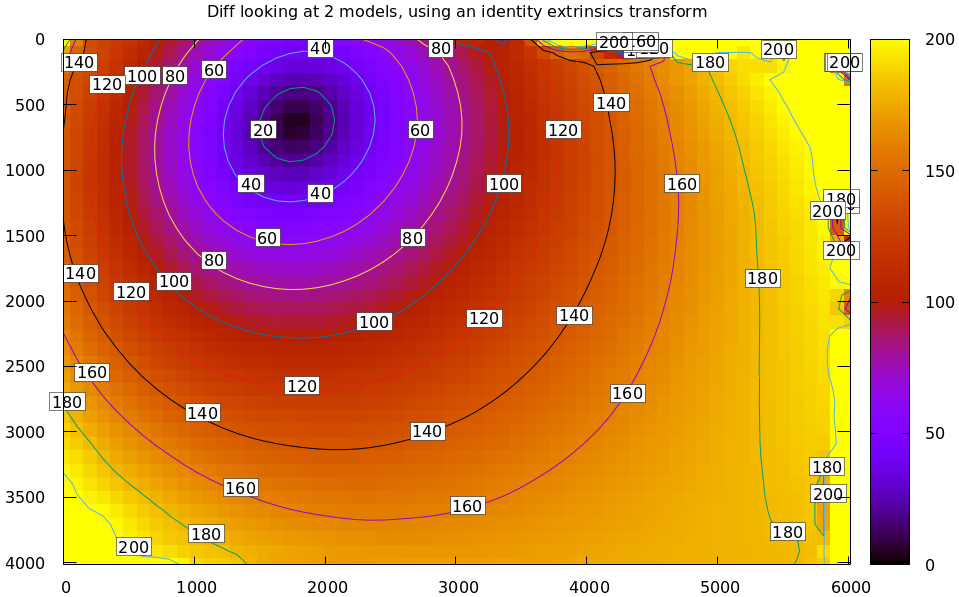And as a vector field:

mrcal-show-projection-diff --radius 0 --cbmax 200 --unset key          \
--vectorfield --vectorscale 5 --gridn 30 20 \
data/board/opencv8.cameramodel
data/board/splined.cameramodel


The reported differences are in pixels.

The issue is similar to the one encountered by the projection uncertainty routine: each calibration produces noisy estimates of all the intrinsics and all the coordinate transformations:

The above plots projected the same $$\vec p$$ in the camera coordinate system, but that coordinate system has shifted between the two models we're comparing. So in the fixed coordinate system attached to the camera housing, we weren't in fact projecting the same point.

There exists some transformation between the camera coordinate system from the solution and the coordinate system defined by the physical camera housing. It is important to note that this implied transformation is built-in to the intrinsics. Even if we're not explicitly optimizing the camera pose, this implied transformation is still something that exists and moves around in response to noise. Rich models like the splined stereographic models are able to encode a wide range of implied transformations, but even the simplest models have some transform that must be compensated for.

The above vector field suggests that we need to move one of the cameras up and to the left, and then we need to rotate that camera. We can automate this by adding a critical missing step to the procedure above between steps 1 and 2:

• Transform $$\vec p$$ from the coordinate system of one camera to the coordinate system of the other camera

We don't know anything about the physical coordinate system of either camera, so we do the best we can: we compute a fit. The "right" transformation will transform $$\vec p$$ in such a way that the reported mismatches in $$\vec q$$ will be minimized.

Once we compute that transformation, and use it to recompute the above diff, we get what we expect:

mrcal-show-projection-diff --unset key                    \
data/board/opencv8.cameramodel \
data/board/splined.cameramodel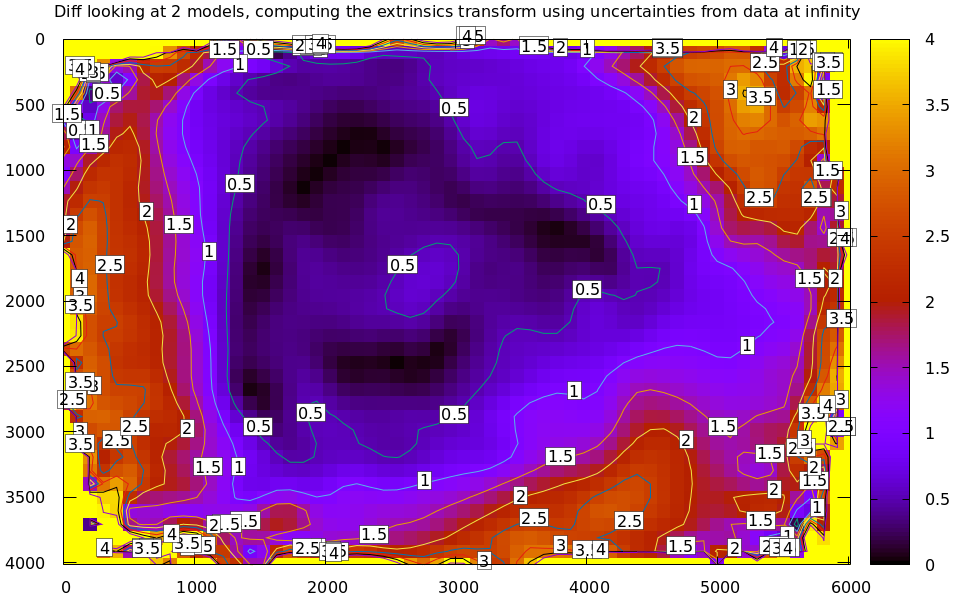## Implied transformation details

As with projection uncertainty, the difference computations are not invariant to range. So we always compute "the projection difference when looking out to $$r$$ meters" for some possibly-infinite $$r$$. The procedure we implement is:

1. Regularly sample the imager, and unproject using lens 0 to get a set of unit direction vectors $$\left\{\vec v_{0_i}\right\}$$ in the camera-0 coordinate system. The mrcal.sample_imager_unproject() does this exactly
2. Scale the direction vectors by the range we care about: $$\left\{\vec p_{0_i}\right\} = \left\{r \vec v_{0_i}\right\}$$. These are now a set of points in the camera-0 coordinate system
3. Compute corresponding unit direction vectors $$\left\{\vec v_{1_i}\right\}$$ in the camera-1 coordinate system
4. Compute the implied transformation $$\left(R,t\right)$$ as the one to maximize $\sum_i w_i \vec v_{1_i}^T \frac{R \vec p_{0_i} + t}{\left|R \vec p_{0_i} + t\right|}$ where $$\left\{w_i\right\}$$ is a set of weights. As with main calibration optimization, this one is unconstrained, using the rt transformation representation. The inner product above is $$\cos \theta$$ where $$\theta$$ is the angle between the two observation vectors.

When looking out to infinity the $$t$$ becomes insignificant, and we do a rotation-only optimization.

This is the logic behind mrcal.implied_Rt10__from_unprojections() and mrcal.projection_diff().

### Selection of fitting data

The idea of using a fit to compute the implied transformation only works when the differences we're seeking are relatively small: once the $$\left(R,t\right)$$ are found, all the fit residuals are low. In many cases this is not a valid assumption. Example: we're comparing two models that both represent a wide lens, except one of the lens models doesn't support wide lenses, so it only fits near the center. In this case, fitting observations everywhere in the imager will include poisoned data off center, so the optimal $$\left(R,t\right)$$ will fit badly. And the reported diff will be thus be high everywhere. However, if the dataset used for the fit is cut down to contain only those observations near the center of the imager, then we will see the effect we expect: the two models would agree in the middle, and diverge at the edges.

Let's demonstrate this. I re-ran the calibration from the tour of mrcal using LENSMODEL_OPENCV4. This model is not expected to work with wide lenses such as this one. But the outlier rejection logic kicks in, makes the solve work as well as it can:

$mrcal-calibrate-cameras \ --corners-cache corners.vnl \ --lensmodel LENSMODEL_OPENCV4 \ --focal 1700 \ --object-spacing 0.077 \ --object-width-n 10 \ --observed-pixel-uncertainty 2 \ --explore \ '*.JPG' vvvvvvvvvvvvvvvvvvvv initial solve: geometry only ^^^^^^^^^^^^^^^^^^^^ RMS error: 32.19393243308935 vvvvvvvvvvvvvvvvvvvv initial solve: geometry and intrinsic core only ^^^^^^^^^^^^^^^^^^^^ RMS error: 12.308083539621906 =================== optimizing everything except board warp from seeded intrinsics mrcal.c(5042): Threw out some outliers (have a total of 491 now); going again mrcal.c(5042): Threw out some outliers (have a total of 894 now); going again ..... a whole lot more of these mrcal.c(5042): Threw out some outliers (have a total of 6764 now); going again mrcal.c(5042): Threw out some outliers (have a total of 6801 now); going again vvvvvvvvvvvvvvvvvvvv final, full re-optimization call to get board warp mrcal.c(5042): Threw out some outliers (have a total of 6831 now); going again ^^^^^^^^^^^^^^^^^^^^ RMS error: 1.6712440499133436 RMS reprojection error: 1.7 pixels Worst residual (by measurement): 8.7 pixels Noutliers: 6831 out of 18600 total points: 36.7% of the data calobject_warp = [-0.00115528 0.00043701] Wrote ./camera-0.cameramodel  The resulting model is available here. This will illustrate the differencing logic, but it isn't a good way to run calibrations, in general: the outlier rejection will throw away the clearly-ill-fitting measurements, but the marginal measurements will make it through, which will produce marginal results. Let's compute the diff between the narrow-only LENSMODEL_OPENCV4 lens model and the mostly-good-everywhere LENSMODEL_OPENCV8 model, using an expanding radius of points. We expect this to work well when using a small radius, and we expect the difference to degrade as we use more and more data away from the center. # This is a zsh loop for r (200 1000 2000 2500) { mrcal-show-projection-diff --no-uncertainties \ --distance 1 --radius$r \
--unset key --extratitle "radius \$r" opencv.cameramodel
}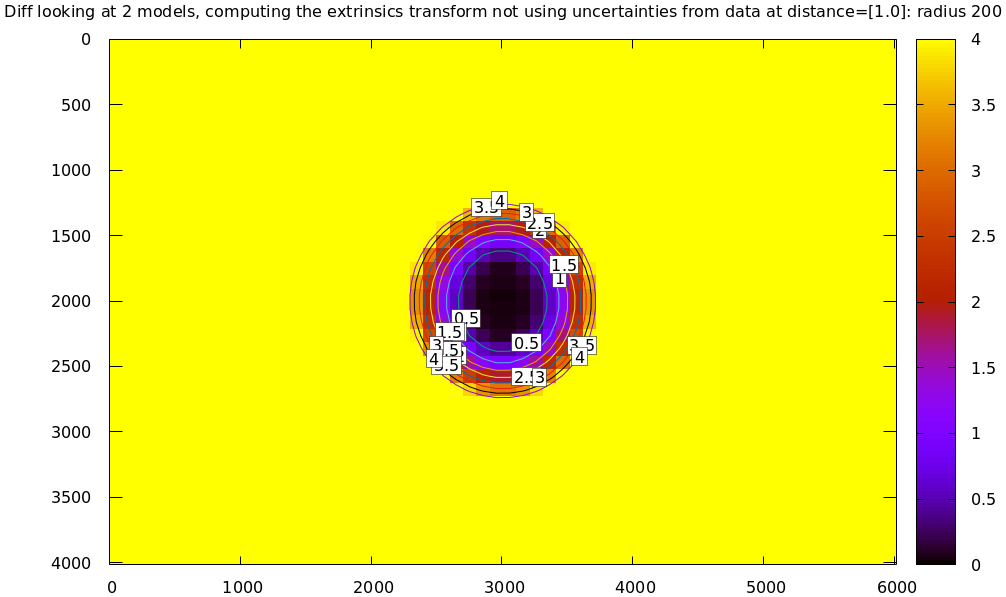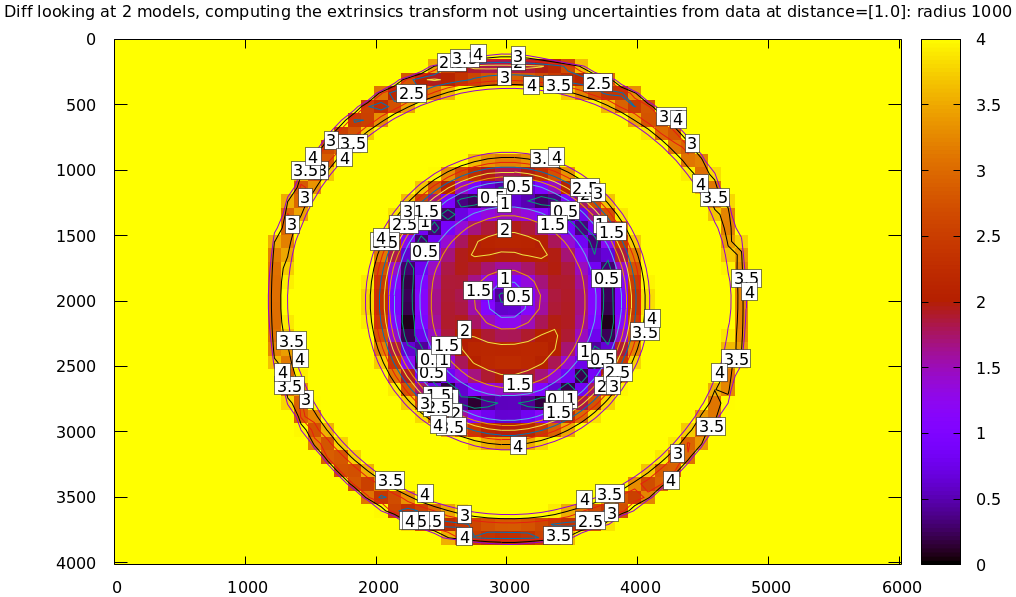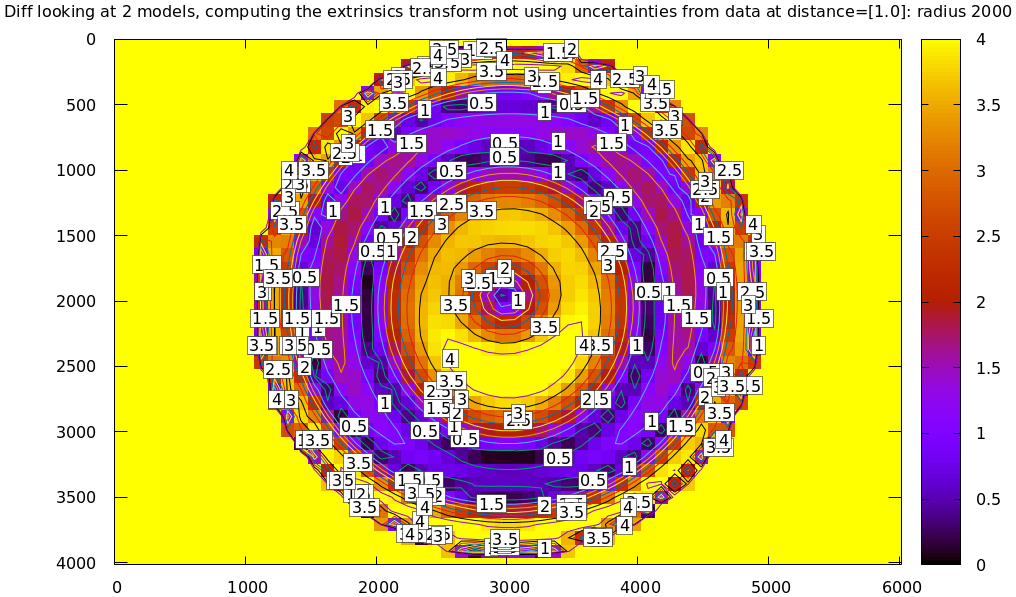### Fit weighting

Clearly the LENSMODEL_OPENCV4 solve does agree with the LENSMODEL_OPENCV8 solve well, but only in the center of the imager. The issue from a tooling standpoint is that in order for the tool to tell us that, we needed to tell the tool to only look at the center. That is not very useful.

This is a work in progress, and mrcal currently has only a partial solution. The problem we observed is that some regions of the imager have unreliable behavior, and poison the fit. But we know where the fit is reliable: in the areas where the projection uncertainty is low. So we can weigh the fit by the inverse of the projection uncertainty, and we will then automatically use the "good" regions while ignoring the "bad" regions. Without requiring the user to specify the good-projection region.

This works, but with a big caveat. As described on the projection uncertainty page, lean models report overly-optimistic uncertainties. Thus when used as weights for the fit, areas that actually are unreliable will be weighted too highly, and will still poison the fit. We see that here, when comparing the LENSMODEL_OPENCV4 and LENSMODEL_OPENCV8 results. The above plots show that the LENSMODEL_OPENCV4 result is only reliable within a few 100s of pixels around the center. However, LENSMODEL_OPENCV4 is a very lean model, so its uncertainty at 1m out (near the sweet spot, where the chessboards were) looks far better than that:

mrcal-show-projection-uncertainty opencv4.cameramodel --distance 1 --unset key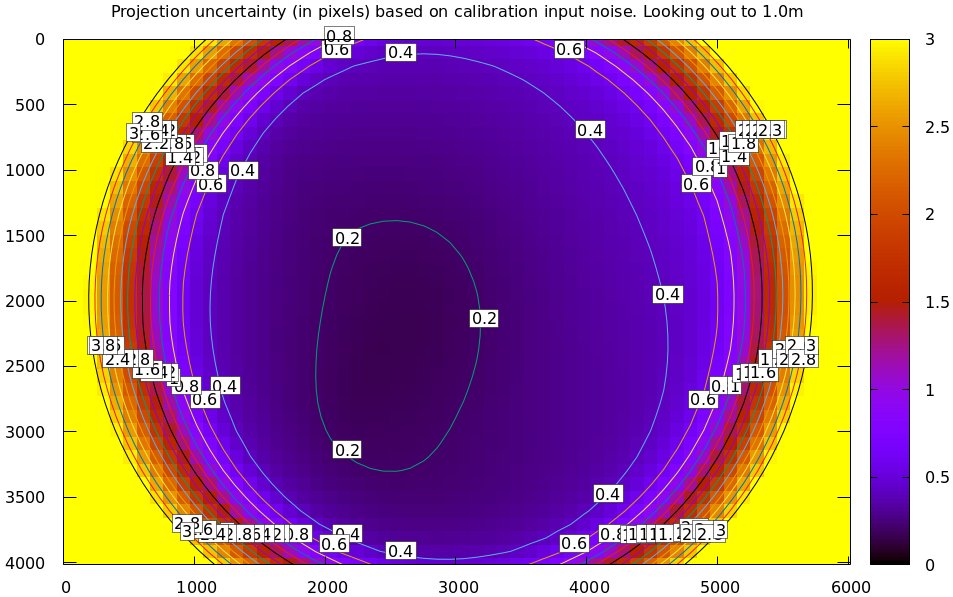And the diff using that uncertainty as a weight without specifying a radius looks poor:

mrcal-show-projection-diff  \
--distance 1
--unset key opencv.cameramodel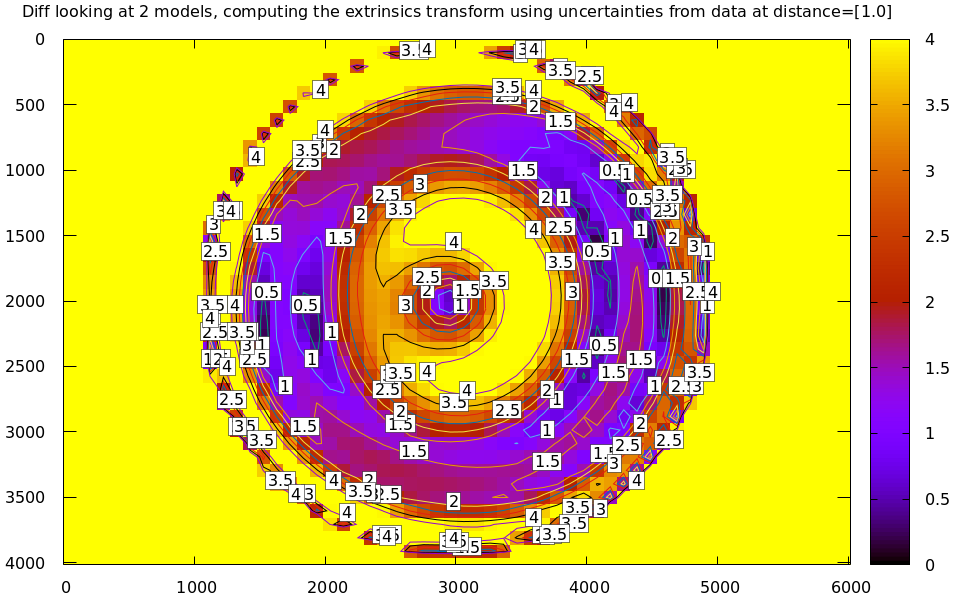Where this technique does work well is when using splined models, which produce realistic uncertainty estimates. To demonstrate, let's cut down the tour of mrcal calibration data to throw out all points outside of a circle at the center, calibrate off that data, and run a diff on those results:

< corners.vnl \
mrcal-cull-corners --imagersize 6016 4016 --cull-rad-off-center 1500 \
> /tmp/raw.vnl &&
vnl-join --vnl-sort - -j filename /tmp/raw.vnl \
<(< /tmp/raw.vnl vnl-filter -p filename --has level |
vnl-uniq -c |
vnl-filter 'count > 20' -p filename ) \
> corners-rad1500.vnl

mrcal-calibrate-cameras               \
--corners-cache corners-rad1500.vnl \
--lensmodel LENSMODEL_OPENCV4       \
--focal 1700                        \
--object-spacing 0.077              \
--object-width-n 10                 \
--observed-pixel-uncertainty 2      \
--explore                           \
'*.JPG'

mrcal-show-projection-uncertainty splined-rad1500.cameramodel \
--distance 1 --unset key

mrcal-show-projection-diff  \
--distance 1
--unset key splined{,-rad1500}.cameramodel


The cut-down corners are here and the resulting model is here. The uncertainty of this model looks like this: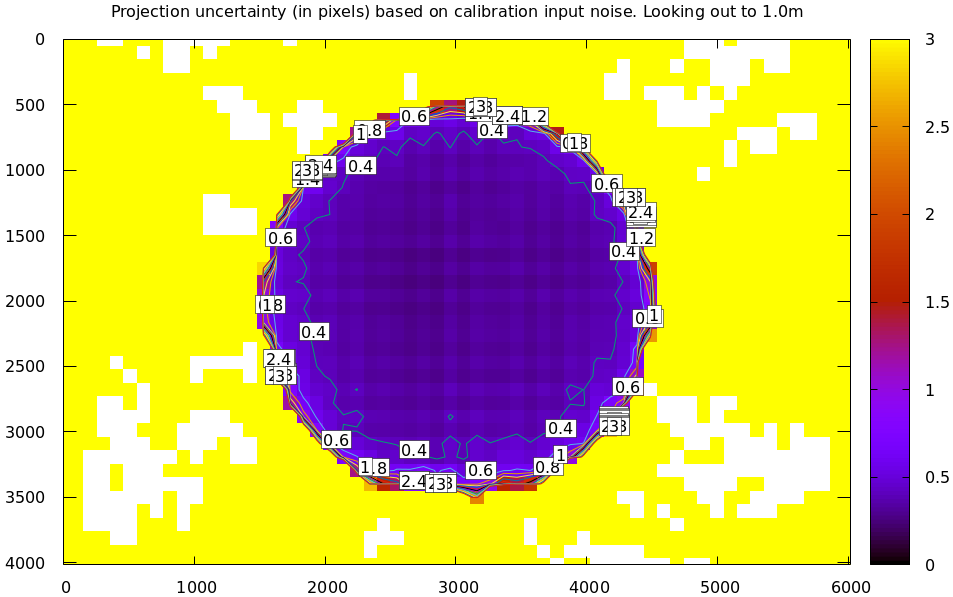and the diff like this: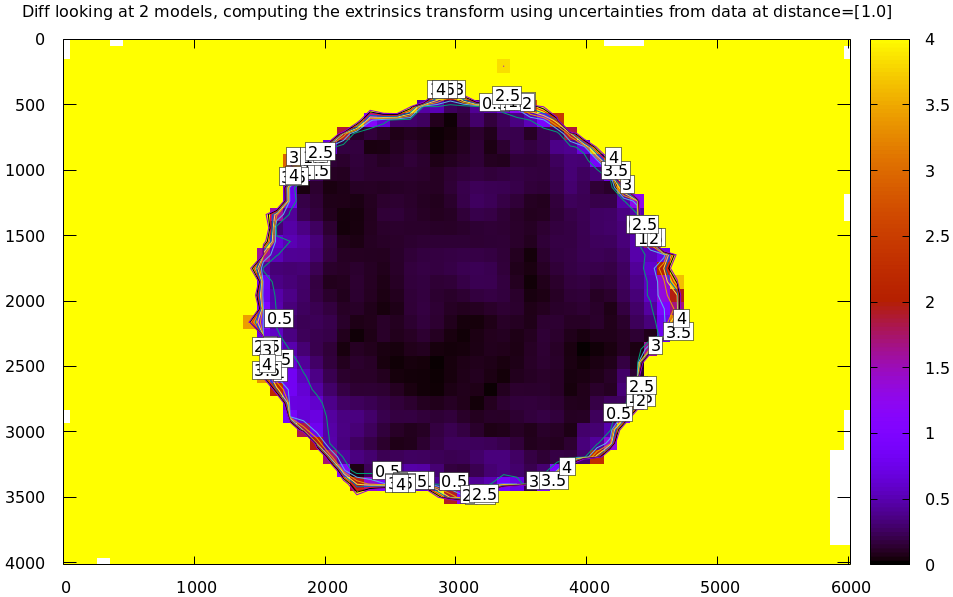## Planned improvements

Various details about the fitting of the implied transformations don't work well, as shown above. Finding better ways to do this would be nice. Potentially we should compute the implied transformation at many ranges at the same time. This needs study.